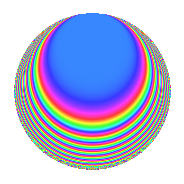# Properties

 Label 4032.2.i.bLevel 4032 Weight 2 Character orbit 4032.i Analytic conductor 32.196 Analytic rank 0 Dimension 8 CM No Inner twists 8

# Related objects

## Newspace parameters

 Level: $$N$$ = $$4032 = 2^{6} \cdot 3^{2} \cdot 7$$ Weight: $$k$$ = $$2$$ Character orbit: $$[\chi]$$ = 4032.i (of order $$2$$ and degree $$1$$)

## Newform invariants

 Self dual: No Analytic conductor: $$32.195682095$$ Analytic rank: $$0$$ Dimension: $$8$$ Coefficient field: 8.0.40960000.1 Coefficient ring: $$\Z[a_1, \ldots, a_{23}]$$ Coefficient ring index: $$2^{8}$$ Sato-Tate group: $\mathrm{SU}(2)[C_{2}]$

## $q$-expansion

Coefficients of the $$q$$-expansion are expressed in terms of a basis $$1,\beta_1,\ldots,\beta_{7}$$ for the coefficient ring described below. We also show the integral $$q$$-expansion of the trace form.

 $$f(q)$$ $$=$$ $$q -\beta_{1} q^{5} + ( -\beta_{2} - \beta_{4} ) q^{7} +O(q^{10})$$ $$q -\beta_{1} q^{5} + ( -\beta_{2} - \beta_{4} ) q^{7} -\beta_{3} q^{11} -2 \beta_{2} q^{17} + 2 \beta_{7} q^{19} -\beta_{6} q^{23} + q^{25} -\beta_{7} q^{29} + 4 \beta_{4} q^{31} + ( -2 \beta_{3} + \beta_{5} ) q^{35} + \beta_{5} q^{37} -2 \beta_{2} q^{41} + 2 \beta_{1} q^{43} + 8 q^{47} + ( 3 - 2 \beta_{6} ) q^{49} -3 \beta_{7} q^{53} + 2 \beta_{4} q^{55} -2 \beta_{5} q^{59} + 6 \beta_{3} q^{61} -\beta_{1} q^{67} -3 \beta_{6} q^{71} + 2 \beta_{6} q^{73} + ( \beta_{1} - \beta_{7} ) q^{77} -4 \beta_{2} q^{79} + 2 \beta_{5} q^{85} + 6 \beta_{2} q^{89} -4 \beta_{6} q^{95} -2 \beta_{6} q^{97} +O(q^{100})$$ $$\operatorname{Tr}(f)(q)$$ $$=$$ $$8q + O(q^{10})$$ $$8q + 8q^{25} + 64q^{47} + 24q^{49} + O(q^{100})$$

Basis of coefficient ring in terms of a root $$\nu$$ of $$x^{8} + 7 x^{4} + 1$$:

 $$\beta_{0}$$ $$=$$ $$1$$ $$\beta_{1}$$ $$=$$ $$($$$$2 \nu^{6} + 16 \nu^{2}$$$$)/3$$ $$\beta_{2}$$ $$=$$ $$($$$$2 \nu^{4} + 7$$$$)/3$$ $$\beta_{3}$$ $$=$$ $$($$$$2 \nu^{7} - \nu^{5} + 13 \nu^{3} - 5 \nu$$$$)/3$$ $$\beta_{4}$$ $$=$$ $$($$$$-2 \nu^{7} - \nu^{5} - 13 \nu^{3} - 5 \nu$$$$)/3$$ $$\beta_{5}$$ $$=$$ $$2 \nu^{6} + 12 \nu^{2}$$ $$\beta_{6}$$ $$=$$ $$($$$$4 \nu^{7} + \nu^{5} + 29 \nu^{3} + 11 \nu$$$$)/3$$ $$\beta_{7}$$ $$=$$ $$($$$$-4 \nu^{7} + \nu^{5} - 29 \nu^{3} + 11 \nu$$$$)/3$$
 $$1$$ $$=$$ $$\beta_0$$ $$\nu$$ $$=$$ $$($$$$\beta_{7} + \beta_{6} + \beta_{4} + \beta_{3}$$$$)/4$$ $$\nu^{2}$$ $$=$$ $$($$$$-\beta_{5} + 3 \beta_{1}$$$$)/4$$ $$\nu^{3}$$ $$=$$ $$($$$$-\beta_{7} + \beta_{6} + 2 \beta_{4} - 2 \beta_{3}$$$$)/2$$ $$\nu^{4}$$ $$=$$ $$($$$$3 \beta_{2} - 7$$$$)/2$$ $$\nu^{5}$$ $$=$$ $$($$$$-5 \beta_{7} - 5 \beta_{6} - 11 \beta_{4} - 11 \beta_{3}$$$$)/4$$ $$\nu^{6}$$ $$=$$ $$($$$$4 \beta_{5} - 9 \beta_{1}$$$$)/2$$ $$\nu^{7}$$ $$=$$ $$($$$$13 \beta_{7} - 13 \beta_{6} - 29 \beta_{4} + 29 \beta_{3}$$$$)/4$$

## Character Values

We give the values of $$\chi$$ on generators for $$\left(\mathbb{Z}/4032\mathbb{Z}\right)^\times$$.

 $$n$$ $$127$$ $$577$$ $$1793$$ $$3781$$ $$\chi(n)$$ $$1$$ $$-1$$ $$-1$$ $$-1$$

## Embeddings

For each embedding $$\iota_m$$ of the coefficient field, the values $$\iota_m(a_n)$$ are shown below.

For more information on an embedded modular form you can click on its label.

Label $$\iota_m(\nu)$$ $$a_{2}$$ $$a_{3}$$ $$a_{4}$$ $$a_{5}$$ $$a_{6}$$ $$a_{7}$$ $$a_{8}$$ $$a_{9}$$ $$a_{10}$$
1889.1
 −0.437016 − 0.437016i 0.437016 + 0.437016i 1.14412 + 1.14412i −1.14412 − 1.14412i 0.437016 − 0.437016i −0.437016 + 0.437016i −1.14412 + 1.14412i 1.14412 − 1.14412i
0 0 0 2.00000i 0 −2.23607 1.41421i 0 0 0
1889.2 0 0 0 2.00000i 0 −2.23607 + 1.41421i 0 0 0
1889.3 0 0 0 2.00000i 0 2.23607 1.41421i 0 0 0
1889.4 0 0 0 2.00000i 0 2.23607 + 1.41421i 0 0 0
1889.5 0 0 0 2.00000i 0 −2.23607 1.41421i 0 0 0
1889.6 0 0 0 2.00000i 0 −2.23607 + 1.41421i 0 0 0
1889.7 0 0 0 2.00000i 0 2.23607 1.41421i 0 0 0
1889.8 0 0 0 2.00000i 0 2.23607 + 1.41421i 0 0 0
 $$n$$: e.g. 2-40 or 990-1000 Embeddings: e.g. 1-3 or 1889.8 Significant digits: Format: Complex embeddings Normalized embeddings Satake parameters Satake angles

## Inner twists

Char. orbit Parity Mult. Self Twist Proved
1.a Even 1 trivial yes
8.b Even 1 yes
12.b Even 1 yes
21.c Even 1 yes
24.f Even 1 yes
28.d Even 1 yes
56.e Even 1 yes
168.i Even 1 yes

## Hecke kernels

This newform can be constructed as the intersection of the kernels of the following linear operators acting on $$S_{2}^{\mathrm{new}}(4032, [\chi])$$:

 $$T_{5}^{2} + 4$$ $$T_{47} - 8$$